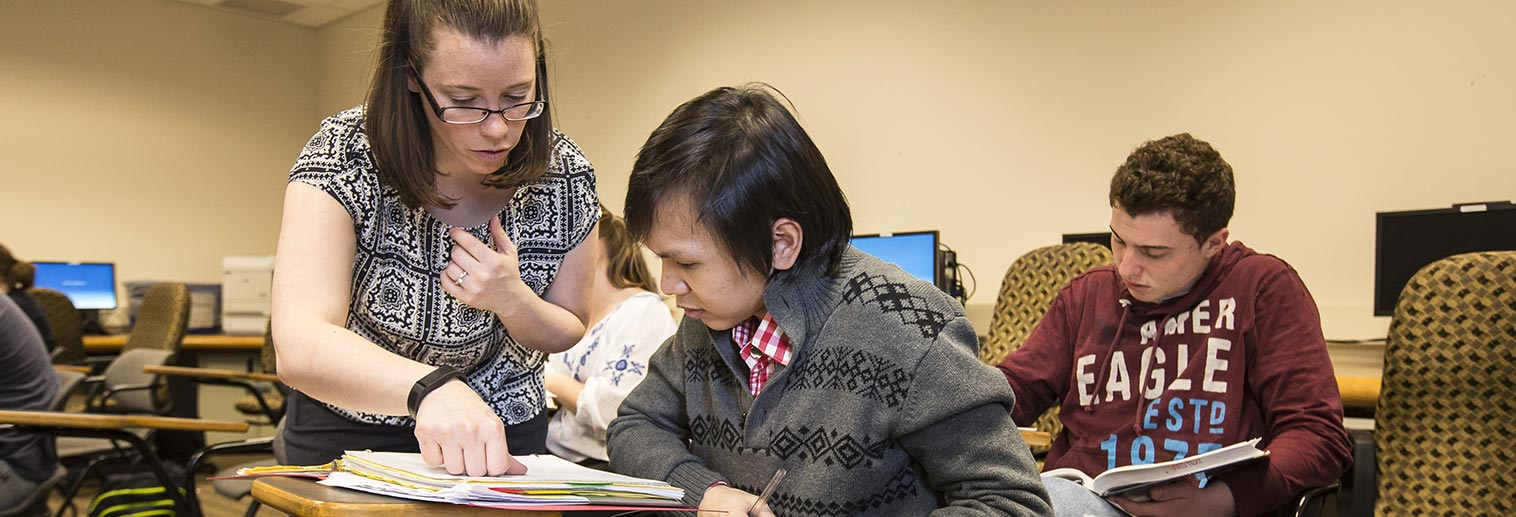Mathematics

Mission, Goals, Outcomes

Mission

The Mathematics program at Moravian College fosters a community of faculty and students who promote the aesthetic, theoretic, and pragmatic qualities of mathematics in order to develop in its students communication and problem solving skills applicable to many disciplines that prepare them for fulfilling careers.

Goals

The Mathematics and Computer Science department has identified the following goals for students in the Mathematics program:

• Mathematical Knowledge - Students will demonstrate broad knowledge of mathematical subject areas, including fundamental concepts as well as the interconnections between various areas of mathematics. In addition, students will recognize connections of mathematics to other disciplines and will be able to solve real problems.
• Problem Solving - Students will demonstrate an ability to solve mathematical problems, making effective use of analytic skills and technology. In doing so, they will demonstrate a willingness to experiment and an ability to represent ideas in multiple ways.
• Communicating Mathematics - Students will develop skills in communicating mathematics; this includes reading, writing and speaking. In all cases, students will show an awareness of context, the notion of proof, and knowledge of notation and terminology.

Outcomes

• Read and demonstrate comprehension of new mathematical material
• Write mathematics with awareness of audience, mathematical context, and proper notation and terminology
• Model a significant real-world problem and solve it using mathematical techniques
• Demonstrate awareness of the role specific mathematical concepts play in several areas of mathematics
• Use Maple to solve mathematical problems by experimenting to gain insight and working through unwieldy computations
• Create and communicate mathematical proofs